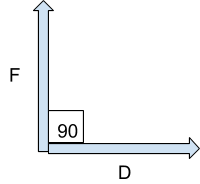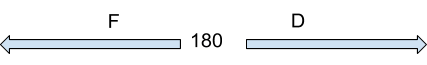# Work Calculator Physics

Enter values in input box to calcualte Work/Force/Distance using this Work done Calculator.

Formula Work = Force * Distance## Formula

Work :
W = Fd (work = force x distance)

Force :
F = W/d

Distance :
d = W/F

Where,
W =Work,
F = Force,
D = Distance.

Work is defined as the integral of the force over a distance of displacement. Work refers to an activity in which one exerts strength or power to do or perform something.

In science, work is said to be done on an object when you transfer energy to that object.

Work formula physics

Work equation or formula in physics:

W = Fd

(work = force x distance)

Force : F = W/d

Distance : d = W/F

Work is also given by the scalar dot product of force and displacement.

W= FDCos

## SI unit of work

The SI unit of work is Joule (J). Here joule is defined as the work done by a force of one newton acting over a distance of one meter. SI base unit of work is kgm2s-2.

In short, work is the amount of energy transferred by a force. Work calculator physics is also known as a joules calculator. Work is denoted by the symbol 'W'.

Work refers to the motion that causes against a resisting force and measured by the product of the force into the component of the motion resolved along the direction of the force. Its dimension is ML2T-2.

## Types of work

There are three types of work.

• Positive (+) work done
• Zero (0) work done
• Negative (-) work done

Positive work done: When force and displacement are in the same direction, work done is positive. Or when the value of theta is between 0 to 90 degrees. E.g pushing or pulling of a door e.t.c.

Zero work done: When force and displacement are normal to each other, work done on the body is Zero. Or when the value of theta is 90 degrees. E.g when you move while holding a bucket full of water e.t.c.Negative work done: When force and displacement are in opposite directions, work done is negative. Or when the value of theta is between 90 to 180 degrees. E.g work done on a ball moving upward due to gravity.## How to calculate work done?

If you are wondering how to find work done manually then below is the guide for you.

1.  Calculate force using the force formula or our online force calculator. Force formula is           F= ma.
2.  Measure angle.
3.  Convert values into the SI unit.
4.  Put the values in the work done equation and solve it.

Example:

Calculate the Work for the given details of an object.

Force (F) = 25 N

Displacement (D) = 10 m

Angle () = 0

Solution:

Apply Work done Formula:

W = Fd Cos

W = 25*10*1

W = 250 J

Work (W) = 250 Joules

## What is the work calculator?

If you are having any problem while calculating work or you still don’t understand how to calculate work done? then don’t worry! This online physics work calculator is used to calculate work done by an object on the other when force and distance are known.

## Daily life examples

The phenomenon of work in physics is the same as in the English language. When we put some effort, we do work. We are cleaning the floor by moving the mop forward and backward, we are doing work. We are piling up some packages on top of each other, work is being done. We are playing badminton, energy is being transferred to the racket, we did work. Hence we are continuously doing some amount of work in our daily life.

1. Work (physics). En.wikipedia.org
2. Work – The Physics Hypertextbook.
3. Work | Definition, Formula, & Units. Encyclopedia Britannica